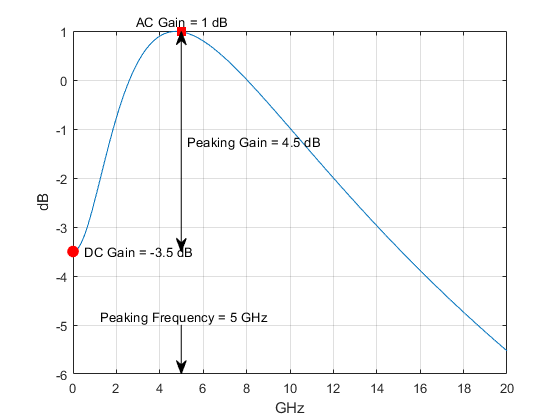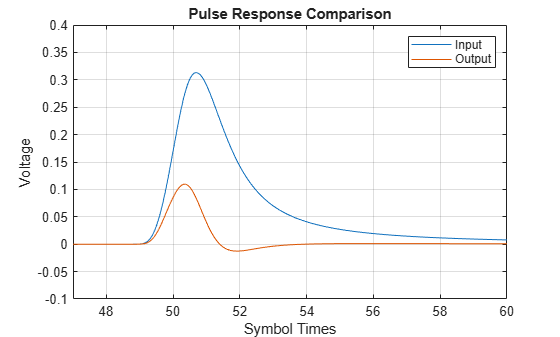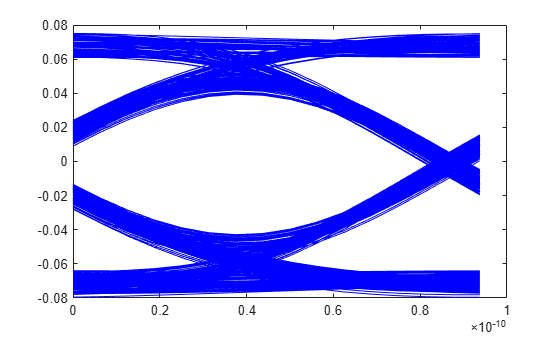# serdes.CTLE

Continuous time linear equalizer (CTLE) or peaking filter

## Description

The `serdes.CTLE` System object™ applies a linear peaking filter to equalize a sample-by-sample input signal or to analytically process an impulse response vector input signal. The equalization process reduces distortions resulting from lossy channels. The filter is a real one-zero two-pole (1z/2p) filter, unless you define the gain-pole-zero (GPZ) matrix.

To equalize the baseband signal using `serdes.CTLE`:

1. Create the `serdes.CTLE` object and set its properties.

2. Call the object with arguments, as if it were a function.

## Creation

### Syntax

``ctle = serdes.CTLE``
``ctle = serdes.CTLE(Name,Value)``

### Description

````ctle = serdes.CTLE` returns a CTLE object that modifies an input waveform according to the pole zero transfer function defined in the object.```
````ctle = serdes.CTLE(Name,Value)` sets properties using one or more name-value pairs. Enclose each property name in quotes. Unspecified properties have default values.Example: `ctle = serdes.CTLE('ACGain',5)` returns a CTLE object with gain at the peaking frequency set to 5 dB.```

## Properties

expand all

Unless otherwise indicated, properties are nontunable, which means you cannot change their values after calling the object. Objects lock when you call them, and the `release` function unlocks them.

If a property is tunable, you can change its value at any time.

### Main

CTLE operating mode, specified as `0`, `1`, or `2`. `Mode` determines whether the CTLE is bypassed or not. If CTLE is not bypassed, then `Mode` also determines what transfer function is applied to the input waveform.

Mode ValueCTLE ModeCTLE Operation
`0`off`serdes.CTLE` is bypassed and the input waveform remains unchanged.
`1`fixed`serdes.CTLE` applies the CTLE transfer function as specified by `ConfigSelect` to the input waveform.
`2`adaptIf `WaveType` is set to `'Impulse'` or `'Waveform'`, then the Init subsystem calls to the `serdes.CTLE`. The `serdes.CTLE` determines the CTLE transfer function to maximize the performance metric as specified by the `PerformanceCriteria` property and applies the transfer function to the input waveform for time domain simulation. This optimized transfer function is used by the CTLE for entire time domain simulation. For more information about the Init subsystem, see Statistical Analysis in SerDes Systems
If `WaveType` is selected as `'Sample'`, then `serdes.CTLE` operates in the fixed mode.

Data Types: `double`

Select which member of the transfer function family to apply in fixed mode, specified as a real integer scalar.

Example: ```ctle = serdes.CTLE('ConfigSelect',5,'Specification','DC Gain and Peaking Gain')``` returns a CTLE object that selects the 6-th element of the `DCGain` and `PeakingGain` vector to apply to the filter transfer function.

Data Types: `double`

Defines which inputs will be used for the CTLE transfer function family. There are five inputs which can be used to define the CTLE transfer function family: DCGain, PeakingGain, ACGain, PeakingFrequency, and GPZ.

You can define the CTLE response from any two of the three gains and peaking frequency or you can define the GPZ matrix for the CTLE.• Select `'DC Gain and Peaking Gain'` to specify CTLE response from `DCGain`, `PeakingGain`, and `PeakingFrequency`.

• `'DC Gain and AC Gain'` to specify CTLE response from `DCGain`, `ACGain`, and `PeakingFrequency`.

• `'AC Gain and Peaking Gain'` to specify CTLE response from `ACGain`, `PeakingGain`, and `PeakingFrequency`.

• `'GPZ Matrix'` to specify CTLE response from `GPZ`.

Data Types: `char`

Approximate frequency at which CTLE transfer function peaks in magnitude, specified as a scalar or a vector in Hz. If specified as a scalar, it is converted to match the length of `ACGain`, `DCGain`, and `PeakingGain` by scalar expansion. If specified as a vector, then the vector length must be the same as the vectors in `ACGain`, `DCGain`, and `PeakingGain`.

Data Types: `double`

Gain at zero frequency for the CTLE transfer function, specified as a scalar or a vector in dB. If specified as a scalar, it is converted to match the length of `PeakingFrequency`, `ACGain`, and `PeakingGain` by scalar expansion. If specified as a vector, then the vector length must be the same as the vectors in `PeakingFrequency`, `ACGain`, and `PeakingGain`.

Data Types: `double`

Peaking gain, specified as a vector in dB. It is the difference between `ACGain` and `DCGain` for the CTLE transfer function. If specified as a scalar, it is converted to match the length of `PeakingFrequency`, `ACGain`, and `DCGain` by scalar expansion. If specified as a vector, then the vector length must be the same as the vectors in `PeakingFrequency`, `ACGain`, and `DCGain`.

Data Types: `double`

Gain at the peaking frequency for the CTLE transfer function, specified as a scalar or vector in dB. If specified as a scalar, it is converted to match the length of `PeakingFrequency`, `DCGain`, and `PeakingGain` by scalar expansion. If specified as a vector, then the vector length must be the same as the vectors in `PeakingFrequency`, `DCGain`, and `PeakingGain`.

Data Types: `double`

Gain pole zero, specified as a matrix. `GPZ` explicitly defines the family of CTLE transfer functions by specifying the `DCGain` (dB) in the first column and then poles and zeros in alternating columns. The poles and zeros are specified in Hz. Additional rows in the matrix define additional configurations, which can be selected using the `ConfigSelect`.

No repeated poles or zeros are allowed. Complex poles or zeros must have conjugates. The number of poles must be greater than number of zeros for system stability. Poles and zeros of 0 Hz is ignored and can be used to zero-pad the matrix.

Data Types: `double`
Complex Number Support: Yes

Time of a single symbol duration, specified as a real scalar in s.

Data Types: `double`

Uniform time step of the waveform, specified as a real scalar in s.

Data Types: `double`

Input wave type form:

• `'Sample'` — A sample-by-sample input signal.

• `'Impulse'` — An impulse response input signal.

• `'Waveform'` — A bit-pattern waveform type of input signal, such as pseudorandom binary sequence (PRBS).

Data Types: `char`

Criterion used for CTLE optimization when the `serdes.CTLE` `Mode` is set to adapt. The performance criteria is calculated using the `optPulseMetric` function.

Data Types: `char`

Method to calculate rational transfer function time domain filter coefficients:

• `PartialFraction` filter method uses a partial fraction expansion of the poles and zeros to directly calculate the filter coefficients.

• `Cascaded` filter method uses an approach that cascades together numerous shorter filters that collectively represent the specified behavior. This results in a more robust filter.

## Usage

### Syntax

``y = ctle(x)``

### Description

example

``y = ctle(x)``

### Input Arguments

expand all

Input baseband signal. If the `WaveType` is set to `'Sample'`, then the input signal is a sample-by-sample signal specified as scalars. If the `WaveType` is set to `'Impulse'`, then the input signal is an impulse response vector signal.

### Output Arguments

expand all

Equalized CTLE output waveform. If the input signal is a sample-by-sample signal specified as scalars, then the output is also scalar. If the input signal is an impulse response vector signal, then the output is also a vector.

## Object Functions

To use an object function, specify the System object as the first input argument. For example, to release system resources of a System object named `obj`, use this syntax:

`release(obj)`

expand all

 `step` Run System object algorithm `release` Release resources and allow changes to System object property values and input characteristics `reset` Reset internal states of System object

## Examples

collapse all

This example shows how to process the impulse response of a channel using `serdes.CTLE` System object™.

Use a symbol time of `100` ps and `16` samples per symbol. The channel has `16` dB loss. The peaking frequency is `11` GHz.

``` SymbolTime = 100e-12; SamplesPerSymbol = 16; dbloss = 16; DCGain = 0:-1:-26; PeakingGain = 0:26; PeakingFrequency = 11e9;```

Calculate the sample interval.

`dt = SymbolTime/SamplesPerSymbol;`

Create the CTLE object. The object adaptively applies the optimum transfer function for the best eye height opening to the input impulse response.

```CTLE1 = serdes.CTLE('SymbolTime',SymbolTime,'SampleInterval',dt,... 'Mode',2,'WaveType','Impulse',... 'DCGain',DCGain,'PeakingGain',PeakingGain,... 'PeakingFrequency',PeakingFrequency);```

Create the channel impulse response.

```channel = serdes.ChannelLoss('Loss',dbloss,'dt',dt,... 'TargetFrequency',1/SymbolTime/2); impulseIn = channel.impulse;```

Process the impulse response with CTLE.

`[impulseOut, Config] = CTLE1(impulseIn);`

`fprintf('Adapted CTLE Configuration Selection is %g \n',Config)`
```Adapted CTLE Configuration Selection is 17 ```

Convert the impulse responses to pulse, waveform, and eye diagram.

```ord = 6; dataPattern = prbs(ord,2^ord-1)-0.5; pulseIn = impulse2pulse(impulseIn,SamplesPerSymbol,dt); waveIn = pulse2wave(pulseIn,dataPattern,SamplesPerSymbol); eyeIn = reshape(waveIn,SamplesPerSymbol,[]); pulseOut = impulse2pulse(impulseOut,SamplesPerSymbol,dt); waveOut = pulse2wave(pulseOut,dataPattern,SamplesPerSymbol); eyeOut = reshape(waveOut,SamplesPerSymbol,[]);```

Create the time vectors.

```t = dt*(0:length(pulseOut)-1)/SymbolTime; teye = t(1:SamplesPerSymbol); t2 = dt*(0:length(waveOut)-1)/SymbolTime;```

Plot pulse response comparison, waveform comparison, input, and output eye diagrams.

```figure plot(t,pulseIn,t,pulseOut) legend('Input','Output') title('Pulse Response Comparison') xlabel('Symbol Times'),ylabel('Voltage') grid on axis([47 60 -0.1 0.4]) ``````figure plot(t2,waveIn,t2,waveOut) legend('Input','Output') title('Waveform Comparison') xlabel('Symbol Times'),ylabel('Voltage') grid on``````figure subplot(211),plot(teye,eyeIn,'b') ax = axis; xlabel('Symbol Times'),ylabel('Voltage') grid on title('Input Eye Diagram') subplot(212),plot(teye,eyeOut,'b') axis(ax); xlabel('Symbol Times'),ylabel('Voltage') grid on title('Output Eye Diagram')```This example shows how to process impulse response of a channel one sample at a time using `serdes.CTLE` System object™.

Use a symbol time of `100` ps and `16` samples per symbol. The channel has `16` dB loss. The peaking frequency is `11 `GHz. Select `12`-th order pseudorandom binary sequence (PRBS), and simulate the first `500` symbols.

```SymbolTime = 100e-12; SamplesPerSymbol = 16; dbloss = 16; DCGain = 0:-1:-26; PeakingGain = 0:26; PeakingFrequency = 11e9; ConfigSelect = 15; prbsOrder = 12; M = 500; ```

Calculate the sample interval.

`dt = SymbolTime/SamplesPerSymbol;`

Create the CTLE object. Since we are processing the channel one sample at a time, the input waveform is `'sample'` type. The object adaptively applies the optimum filter transfer function for the best eye height opening.

```CTLE = serdes.CTLE('SymbolTime',SymbolTime,'SampleInterval',dt,... 'Mode',2,'WaveType','Sample',... 'DCGain',DCGain,'PeakingGain',PeakingGain,... 'PeakingFrequency',PeakingFrequency,... 'ConfigSelect',ConfigSelect);```

Create the channel impulse response.

```channel = serdes.ChannelLoss('Loss',dbloss,'dt',dt,... 'TargetFrequency',1/SymbolTime/2);```

Create the eye diagram.

```eyediagram = comm.EyeDiagram('SampleRate',1/dt,'SamplesPerSymbol',SamplesPerSymbol,... 'YLimits',[-0.5 0.5]);```
```Warning: comm.EyeDiagram will be removed in a future release. Use eyediagram instead. ```

Initialize PRBS generator.

`[dataBit,prbsSeed] = prbs(prbsOrder,1);`

Loop through one symbol at a time.

``` inwave = zeros(SamplesPerSymbol,1); outwave = zeros(SamplesPerSymbol,1); for ii = 1:M % Get new symbol [dataBit,prbsSeed] = prbs(prbsOrder,1,prbsSeed); inwave(1:SamplesPerSymbol) = dataBit-0.5; % Convolve input waveform with channel y = channel(inwave); % Process one sample at a time through the CTLE for jj = 1:SamplesPerSymbol outwave(jj) = CTLE(y(jj)); end % Plot eye diagram eyediagram(outwave) end```## Extended Capabilities